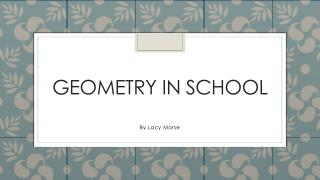DownloadDownload PresentationGeometry in school

Geometry in school

Télécharger la présentationGeometry in school

- - - - - - - - - - - - - - - - - - - - - - - - - - - E N D - - - - - - - - - - - - - - - - - - - - - - - - - - -
Presentation Transcript

1. Geometry in school By Lacy Morse

2. Circle A circle is flat It has no vertices , Edges or faces But this one does

3. Sphere A sphere is like a circle No vertices, edges or faces but it is not flat It is 3-D

4. Rectangle A rectangle has four Right angles it has two Pairs of parallel lines

5. Square A square is a quadrilateral It has four right angles All the sides are the same And it has two pairs of parallel lines

6. cube A cube is 3D It has six faces, Eight vertices, twelve edges a cubes faces are made of squares.

7. triangle A triangle has three Kinds equilateral, isosceles, scalene and a triangles can have Acute angle, obtuse angle, And right angle

8. Cylinder A cylinder has two faces No vertices or edges the Top and the bottom are faces

9. Parallel lines Parallel lines the two bars are parallel because they will never meet

10. Rectangular prism A rectangular prism is a type of pyramid it has five faces eight edges five vertices